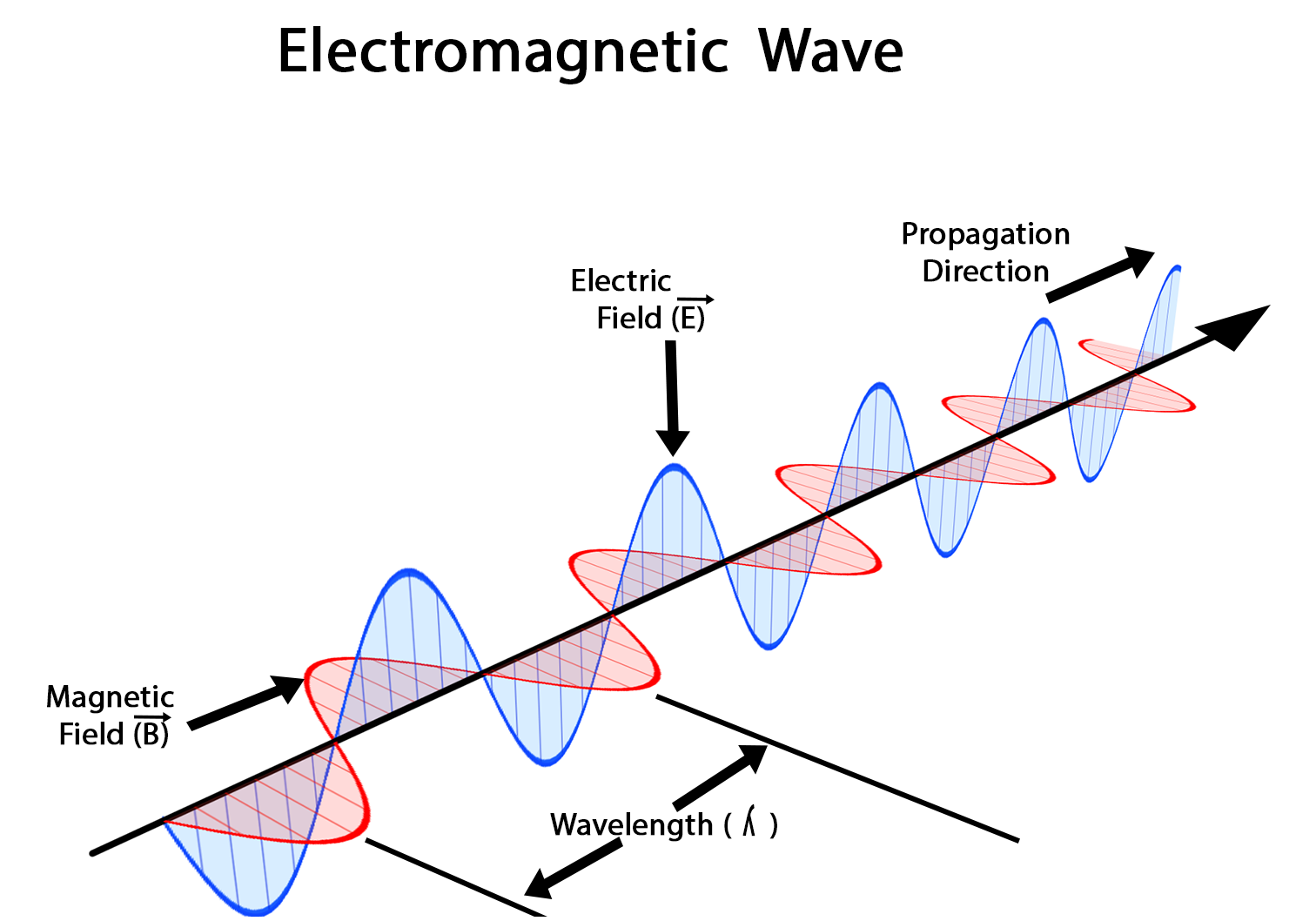# The Start Of The Special Relativity

4년 전

In my last post I mentioned that I would write about special relativity.
First I will cover some background information in order for you to understand the physical theory better.

We already know from my last post that the classical physics cannot explain a phenomenon like in the Michelson Morley Experiment. Because scientists believed that electromagnetic radiations would travel through a substance called aether.

Electromagnetic radiation (EMR) refers to waves in the electromagnetic spectrum and includes light, radio waves, microwaves etc.Why did they believe that there has to be a medium? It is because waves normally need a medium to travel through. An example would be sound waves which need a medium like air to travel. That is why you cannot hear explosions in space, a vacuum.

Again due to the Michelson Morley Experiment, we know that light doesn’t follow the rules of the classical physics. This means we need a new theory because the old one was proven wrong. In fact, there were now many possibilities one was published by Albert Einstein.This is the birth of the special relativity. 1905 Einstein published a paper "Zur Elektrodynamik bewegter Körper" ("On the Electrodynamics of Moving Bodies") which combines Maxwell’s equations with laws of mechanic.

Hold on! What are Maxwell's equations and what laws of mechanic exactly?

Maxwell's equations are a set of 4 equations which explain the way magnetic and electric fields interact with each other. These equations build with the Lorentz force the foundation of classical electromagnetism, classical optics and electrodynamics. It is important to know that magnetic fields are somehow connected to electric fields. This leads to EMRs and these special kind of waves don’t follow the classical rules of physics like mentioned above.

In Einstein’s paper two postulates were formulated:

The principle of relativity: The laws in nature are the same in all inertial reference frames

This means all laws apply the same way and we have to recognize these inertial systems as equal to each other.
An example for an inertial system would be the inertial system Human – Earth and the laws would apply the same way in the inertial system Earth-Galaxy

The Constance of Light: In all inertial systems is the speed of light in a vacuum the same for all observers.

This means that light (EMR) has the same speed and it does not matter whether the light source is moving or not. Light travels in a vacuum with about 2,99792458*10ˆ8 m/s.

A vacuum is space with a devoid of matter. In this space exists a gaseous pressure a pressure which equals zero pressure. There is not such a thing like the ideal vacuum. However, we have to keep in mind that pressure could interact with the speed of light. Even though we are now thinking about differences which are for us imperceptible.

So these are the two postulates Einstein formulated and these postulates had consequences or rules. Consequences such that light travels with the maximal possible speed, consequences like the time dilatation, and the length contraction.
These rules were till now proven to be right and I will mention some experiments in the next post.

As always feel free to correct me. I hope you enjoyed reading. A feedback in the comments would be great.